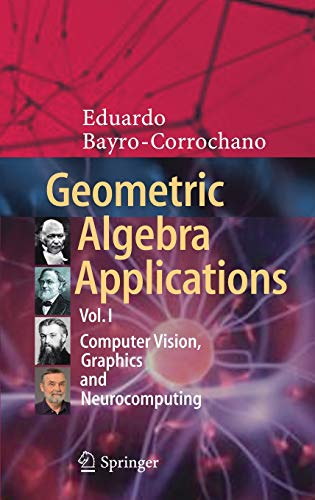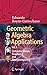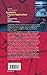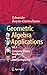Sunday , July 12th 2020

# Geometric Algebra Applications Vol. I: Computer Vision, Graphics and Neurocomputing#### Product Features:

The goal of the Volume I Geometric Algebra for Computer Vision, Graphics  and Neural Computing is to present a unified mathematical treatment of diverse problems in the general domain of artificial intelligence and associated fields using Clifford, or geometric, algebra.Geometric algebra provides a rich and general mathematical framework for…
Price as on: 2020-01-15 15:46:29

#### Product Description

The goal of the Volume I Geometric Algebra for Computer Vision, Graphics  and Neural Computing is to present a unified mathematical treatment of diverse problems in the general domain of artificial intelligence and associated fields using Clifford, or geometric, algebra.

Geometric algebra provides a rich and general mathematical framework for Geometric Cybernetics in order to develop solutions, concepts and computer algorithms without losing geometric insight of the problem in question. Current mathematical subjects can be treated in an unified manner without abandoning the mathematical system of geometric algebra for instance: multilinear algebra, projective and affine geometry, calculus on manifolds, Riemann geometry, the representation of Lie algebras and Lie groups using bivector algebras and conformal geometry.

By treating a wide spectrum of problems in a common language, this Volume I offers both new insights and new solutions that should be useful to scientists, and engineers working in different areas related with the development and building of intelligent machines. Each chapter is written in accessible terms accompanied by numerous examples, figures and a complementary appendix on Clifford algebras, all to clarify the theory and the crucial aspects of the application of geometric algebra to problems in graphics engineering, image processing, pattern recognition, computer vision, machine learning, neural computing and cognitive systems.

## Complexity Theory, Game Theory, and Economics: The

This monograph comprises a series of ten lectures divided into two parts. Part 1, referred to as the Solar…

## Computer Science Education

This book provides an overview of how to approach computer science education research from a pragmatic perspective. It represents…

## Supervised Learning with Quantum Computers (Quantum

Quantum machine learning investigates how quantum computers can be used for data-driven prediction and decision making. The books summarises…